Courses

# Partial Molar Property Civil Engineering (CE) Notes | EduRev

## Civil Engineering (CE) : Partial Molar Property Civil Engineering (CE) Notes | EduRev

The document Partial Molar Property Civil Engineering (CE) Notes | EduRev is a part of the Civil Engineering (CE) Course Thermodynamics.
All you need of Civil Engineering (CE) at this link: Civil Engineering (CE)

Partial Molar Property

We consider first the case of a homogenous (single-phase), open system that can interchange matter with its surroundings and hence undergo a change of composition. Therefore, the total value of any extensive property M t ( M ≡ V ,U , H , S, A,G )  is not only a function of T and P, but also of the actual number of moles of each species present in the system. Thus, we may write the following general property relation:

(Mt = nM = M (T , P, n, n2 …..ni….nN )                   ......(6.1)

Where N ≡ total number of chemical species in t he system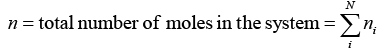......(6.2)

Taking the total derivative for both sides of eqn. 6.1: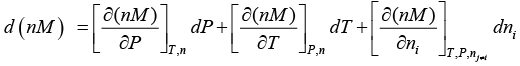......(6.2)

Where, subscript n indicates that all mole numbers are held constant and subscript n j ≠i that all molenumbers except ni are held constant. This equation has the simpler form: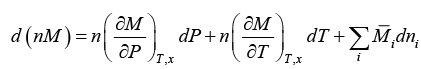......(6.3)

(the subscript x denotes differential at constant composition)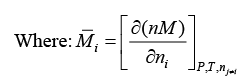......(6.4)

Eqn. 6.4 defines the partial molar property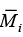of species i in solution. It represents the change of total property ‘nM’ of a mixture resulting from addition at constant T and P of a differential amount of species ‘i’ to a finite amount of solution. In other words it also signifies the value of the property per mole of the specific species when it exists in solution. In general, the partial molar property of a substance differs from the molar property of the same substance in a pure state at the same temperature and pressure as the mixture or solution.  This owing to the fact that while in a pure state the molecules interact with its own species, in a solution it may be subjected to different interaction potential with dissimilar molecules. This may render the value of a molar property different in mixed and pure states.

Now, ni = xi n; dni = xdn + n dxi

Or : dn= x, dn + n dxi                     ......(6.5)

Also: d ( nM ) = ndM + Mdn                           ......(6.6)

Substituting eqns. 6.5 and 6.6 in eqn. 6.3 leads to: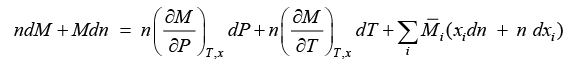......(6.7)

On re-arranging: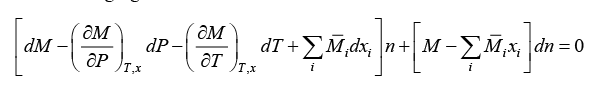......(6.8)

While deriving eqn. 6.8 no specific constraints on the values of either n or dn have been applied. This suggests that the equation is valid for any arbitrary values of these two variables. Thus n and dn are independent of each other. Therefore, eqn. 6.8 can only be valid if the coefficients of these two variables are identically zero. On putting the coefficients to zero the following equations obtain: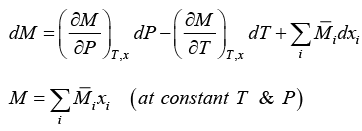......(6.9, 6.10)

From eqn. 6.10, it follows, that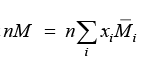......(6.11)

And also: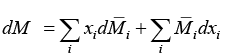......(6.12)

Equating (6.19) and (6.21) yields the well-known Gibbs-Duhem equation (GDE):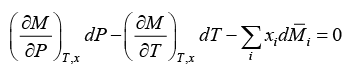......(6.13)

The GDE must be satisfied for all changes in P, T, and in M i caused by changes of state in a homogeneous phase. For the important special case of changes at constant T and P, it simplifies to: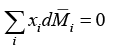......(6.14)

Or, taking any arbitrary species ‘j’: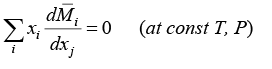......(6.15)

Equations (6.14 and 6.15) implies that the partial molar properties of the various species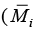's are not independent. Some key properties of partial molar properties are defined as follows: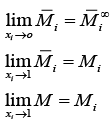Select additional relations among partial properties are demonstrated in the Appendix 6.1. Based on the foregoing considerations one may define an isothermal molar property change of mixing

∆M as follows:

∆M mix (T,P) = M (T,P) −Σxi M i (T,P)                     ......(6.16)

Or: ∆M mix = M −ΣxiMi = ΣxiMi −ΣxiMi = Σxi (M i − M i)                   ......(6.17)

(M can be = V, U, H, S, A, G)

Typical examples of molar volume change of mixing for a number of binary solutions are shown in figs. 6.1 and 6.2. Clearly then there can be substantial variation of this property depending upon the nature of the constituent molecules.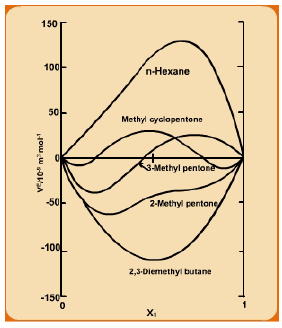Fig. 6.1 Molar volume change of mixing for solutions of cyclohexane (1) with some other C6 hydrocarbons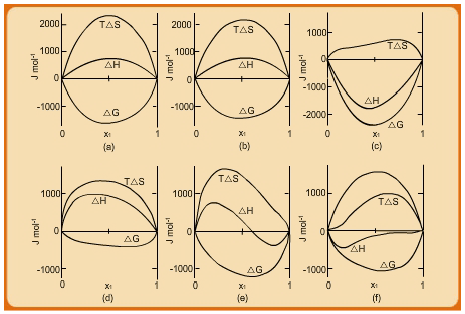Fig. 6.2 Property changes of mixing at 50°C for 6 binary liquid systems: (a) chloroform(1)/nheptane(2); (b) acetone(1)/methanol(2); (c) acetone(1)/chloroform(2); (d) ethanol(1)/n-heptane(2); (e) ethanol(1)/chloroform(2); ( f) ethanol(1)/water(2).

Offer running on EduRev: Apply code STAYHOME200 to get INR 200 off on our premium plan EduRev Infinity!

## Thermodynamics

56 videos|92 docs|33 tests

,

,

,

,

,

,

,

,

,

,

,

,

,

,

,

,

,

,

,

,

,

;